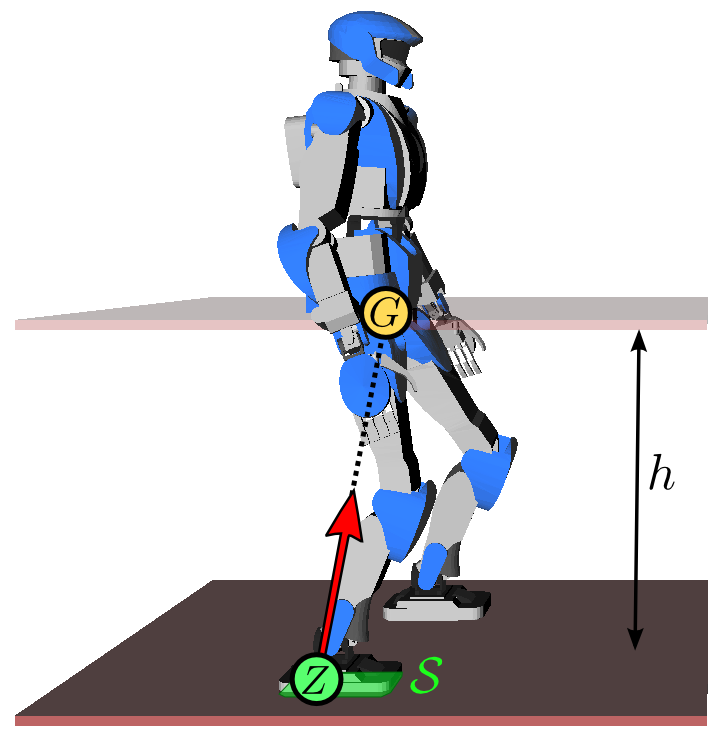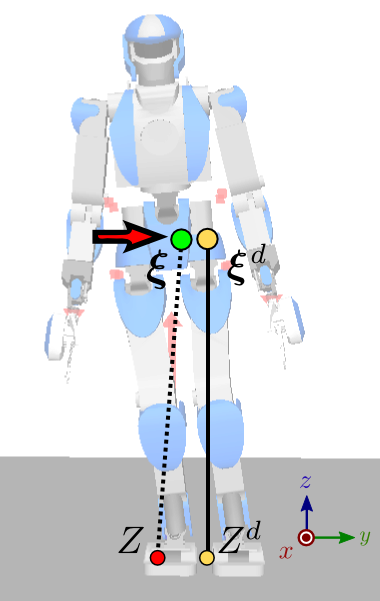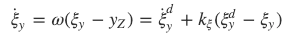# [双足机器人如何行走？]

## 线性倒立摆模型(LIPM)

• 假设 1：机器人有足够的关节力矩来实现其运动；
• 假设 2：质心(CoM)周围没有角动量；
• 假设 3：质心周围没有角动量；

$\ddot{p}_{G}=\omega^{2}\left(p_{G}-p_{Z}\right)$• 步态模式生成：假设一个理想的模型，并且没有干扰，生成参考的 CoM-ZMP 轨迹；
• 步态稳定性：尽可能地去拟合跟踪参考轨迹，使用反馈控制来消除干扰和修正模型误差；

## 步态模式生成

$p_{Z}=p_{G}-\frac{\ddot{p}_{G}}{\omega^{2}}$

ZMP 需要始终处于支撑区 $S$ 内。如果机器人处于单脚支撑时，支撑区就成了单个点。如果机器人在平地上双脚着地，所有支撑区就形成一个凸包形状。如果地面不平，或者机器人由其他接触（例如用手倾斜靠在某处），则定义一个多接触 ZMP 区，但这就会是相当复杂的问题了。

### DCM 轨迹生成

$\xi=p_{G}+\frac{\dot{p}_{G}}{\omega}$

\begin{aligned} \dot{\xi} &=\omega\left(\xi-p_{Z}\right) \\ \dot{p}_{G} &=\omega\left(\xi-p_{G}\right) \end{aligned}

## 步态稳定性

$\dot{\xi}=\dot{\xi}^{d}+k_{\xi}\left(\xi^{d}-\xi\right)$

$p_{Z}=p_{Z}^{d}+k_{z}\left(\xi-\xi^{d}\right)$